1. /
2. CBSE
3. /
4. Class 08
5. /
6. Mathematics
7. /
8. NCERT Solutions for Class...

# NCERT Solutions for Class 8 Maths Exercise 7.1### myCBSEguide App

Download the app to get CBSE Sample Papers 2023-24, NCERT Solutions (Revised), Most Important Questions, Previous Year Question Bank, Mock Tests, and Detailed Notes.

NCERT solutions for Class 8 Maths Cubes and Cube Roots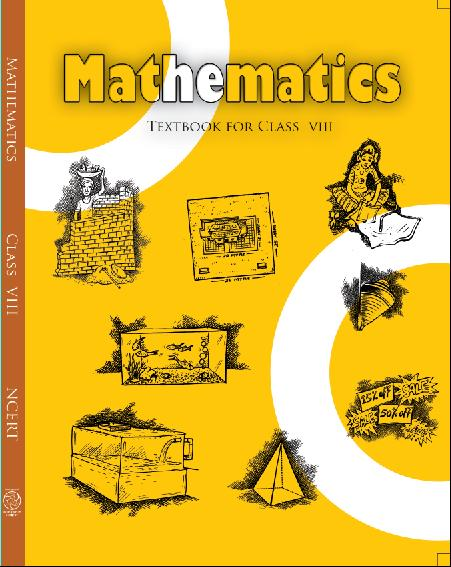## NCERT Solutions for Class 8 Maths Cubes and Cube Roots

###### 1. Which of the following numbers are not perfect cubes:

(i)216

(ii)128

(iii)1000

(iv)100

(v)46656

Ans. (i)216

Prime factors of 216 =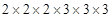Here all factors are in groups of 3’s (in triplets)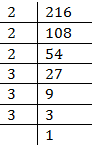Therefore, 216 is a perfect cube number.

(ii) 128

Prime factors of 128

=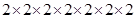Here one factor 2 does not appear in a 3’s group.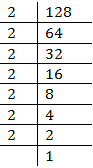Therefore, 128 is not a perfect cube.

(iii) 1000

Prime factors of 1000 =Here all factors appear in 3’s group.

Therefore, 1000 is a perfect cube.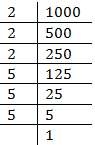(iv) 100

Prime factors of 100 = 2 x 2 x 5 x 5

Here all factors do not appear in 3’s group.

Therefore, 100 is not a perfect cube.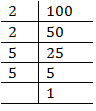(v) 46656

Prime factors of 46656

=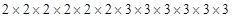Here all factors appear in 3’s group.

Therefore, 46656 is a perfect cube.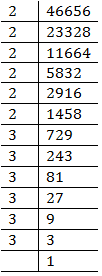NCERT Solutions for Class 8 Maths Exercise 7.1

###### 2. Find the smallest number by which each of the following numbers must be multiplied to obtain a perfect cube:

(i)243

(ii)256

(iii)72

(iv)675

(v)100

Ans. (i)243

Prime factors of 243 =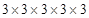Here 3 does not appear in 3’s group.

Therefore, 243 must be multiplied by 3 to make it a perfect cube.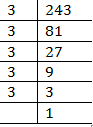###### (ii) 256

Prime factors of 256

=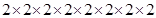Here one factor 2 is required to make a 3’s group.

Therefore, 256 must be multiplied by 2 to make it a perfect cube.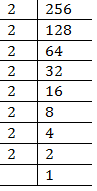(iii) 72

Prime factors of 72 =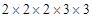Here 3 does not appear in 3’s group.

Therefore, 72 must be multiplied by 3 to make it a perfect cube.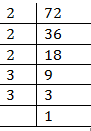(iv) 675

Prime factors of 675 =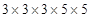Here factor 5 does not appear in 3’s group.

Therefore 675 must be multiplied by 3 to make it a perfect cube.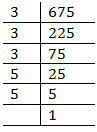(v)  100

Prime factors of 100 =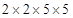Here factor 2 and 5 both do not appear in 3’s group.

Therefore 100 must be multiplied by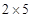= 10 to make it a perfect cube.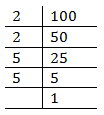NCERT Solutions for Class 8 Maths Exercise 7.1

###### 3. Find the smallest number by which each of the following numbers must be divided to obtain a perfect cube:

(i)81

(ii)128

(iii)135

(iv)192

(v)704

Ans. (i)81

Prime factors of 81 =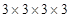Here one factor 3 is not grouped in triplets.

Therefore 81 must be divided by 3 to make it a perfect cube.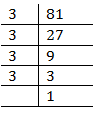(ii) 128

Prime factors of 128 =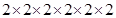Here one factor 2 does not appear in a 3’s group.

Therefore, 128 must be divided by 2 to make it a perfect cube.

######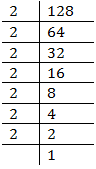(iii) 135

Prime factors of 135 =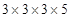Here one factor 5 does not appear in a triplet.

Therefore, 135 must be divided by 5 to make it a perfect cube.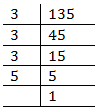(iv) 192

Prime factors of 192 =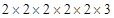Here one factor 3 does not appear in a triplet.

Therefore, 192 must be divided by 3 to make it a perfect cube.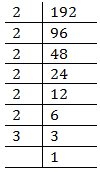(v) 704

Prime factors of 704

=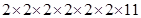Here one factor 11 does not appear in a triplet.

Therefore, 704 must be divided by 11 to make it a perfect cube.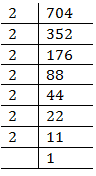NCERT Solutions for Class 8 Maths Exercise 7.1

###### 4. Parikshit makes a cuboid of plasticine of sides 5 cm, 2 cm, 5 cm. How many such cuboids will he need to form a cube?

Ans. Given numbers =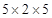Since, Factors of 5 and 2 both are not in group of three.

Therefore, the number must be multiplied by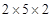= 20 to make it a perfect cube.

Hence he needs 20 cuboids.

## NCERT Solutions for Class 8 Maths Exercise 7.1

NCERT Solutions Class 8 Mathematics PDF (Download) Free from myCBSEguide app and myCBSEguide website. Ncert solution class 8 Mathematics includes text book solutions from Class 8 Maths Book . NCERT Solutions for CBSE Class 8 Maths have total 16 chapters. 8 Maths NCERT Solutions in PDF for free Download on our website. Ncert class 8 solutions PDF and Maths ncert class 8 PDF solutions with latest modifications and as per the latest CBSE syllabus are only available in myCBSEguide.

## CBSE app for Class 8

To download NCERT Solutions for class 8 Social Science, Computer Science, Home Science,Hindi ,English, Maths Science do check myCBSEguide app or website. myCBSEguide provides sample papers with solution, test papers for chapter-wise practice, NCERT solutions, NCERT Exemplar solutions, quick revision notes for ready reference, CBSE guess papers and CBSE important question papers. Sample Paper all are made available through the best app for CBSE students and myCBSEguide website.Test Generator

Create question paper PDF and online tests with your own name & logo in minutes.myCBSEguide

Question Bank, Mock Tests, Exam Papers, NCERT Solutions, Sample Papers, Notes

### 1 thought on “NCERT Solutions for Class 8 Maths Exercise 7.1”

1. very helpful for me in clearing my doubts thanks a lot !